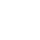hangzhou manba envirnomental protection co.,ltd.

Hangzhou Mamba Environmental Protection Co. , Ltd.

# 你知道为什么碳氮磷比控制在100：5：1吗？

2022-09-15 12:57:46

CNP比100:5:1是怎么来的？
1、CNP比100：5：1的比例是针对于好氧除碳工艺的营养比！而非厌氧与脱氮工艺的CNP比！

2、碳氮磷代表的值，Ｃ目前争议最多，代表COD或者BOD各有支持人群（其实工程应用比较灵活，多了少加，少了多加，营养源的计算只是一个大体的估值，笔者颜胖子个人偏重于COD的说法，这样的计算结果不会使碳源投加过量，毕竟一切服务于实际）；N一般指总凯氏氮（TKN），包括有机氮和氨氮，但不包括亚硝氮和硝态氮；而磷最没有争议性一般为正磷酸盐。

3、100：5：1比例的来源：

BOD：N：P=（52.4%/20%）：12.2%：2.3%=100：5：1。

1、外部碳源投加量简易计算方法

Cm=20N-C （式1）

Cm—必须投加的外部碳源量（以COD计）mg/l；

20—CN比；

N—需要去除的TKN的量，mg/l

C—进出水的碳源差值（以COD计）mg/l

N=Ne-Ns （式2）

Ns—二沉池TKN排放指标mg/l

C=Ce-Cs（式3）

Ce—进水实际COD浓度mg/l；

Cs—二沉池COD排放指标mg/l

2、案例计算

N=Ne-Ns=15-5=10(mgN／L)

C=Ce-Cs=100-10=90mg／L

Cm=20N-C=20×10-90=110(mgCOD/L)

Cd=QCm=1×10^4×110×10^-3=1100(kgCOD/d)

1100/1.07=1028kg/d

1100/1.5=733kg/d

1100/0.78=1410kg/d

1100/1.06=1037kg/d

1、外部碳源投加量简易计算方法

Cm=5N （式4）

Cm—必须投加的外部碳源量（以COD计）mg/l；

5—反硝化1kgNO-3-N需投加外部碳源(以COD计)5kg；

N—需要外部碳源去除的TN量，mg/l

N=Ne-Ns （式5）

Ne—二沉池出水实际TN浓度mg/l；

Ns—二沉池TN排放标准mg/l

2、案例计算

N=Ne-Ns=20-15=5(mgN／L)

Cm=5N=5×5=25(mgCOD/L)

Cd=QCm=1×10^4×25×10^-3=250(kgCOD/d)

250/1.07=233kg/d

250/1.5=166kg/d

250/0.78=320kg/d

250/1.06=235kg/d

1、外部碳源投加量简易计算方法

Cm=15P-C（式6）

Cm—必须投加的外部碳源量（以COD计）mg/l；

15—CP比；

P—需要外部碳源去除的TP量，mg/l

C—进出水的碳源差值（以COD计）mg/l

2、需用外部碳源去除的TP量计算

P=Pe-Ps （式7）

Pe—进水TP浓度mg/l；

Ps—二沉池TP排放标准mg/l

C=Ce-Cs（式8）

Ce—进水实际COD浓度mg/l；

Cs—二沉池COD排放标准mg/l

3、案例计算

P=Pe-Ps=11-1=10(mgN／L)

C=Ce-Cs=100-10=90mg／L

Cm=15P-C=15×10-90=60(mgCOD/L)

Cd=QCm=1×10^4×60×10^-3=600(kgCOD/d)

600/1.07=560kg/d

600/1.5=400kg/d

600/0.78=769kg/d

600/1.06=566kg/d

recommendationrecommendation0571-63789217• 取消“扫一扫”加入群聊